Jan Lorenz (2006)

Consensus Strikes Back in the Hegselmann-Krause Model of Continuous Opinion Dynamics Under Bounded Confidence

Journal of Artificial Societies and Social Simulation vol. 9, no. 1
<https://www.jasss.org/9/1/8.html>

Received: 06-Jun-2005    Accepted: 28-Sep-2005    Published: 31-Jan-2006

###Abstract

The agent-based bounded confidence model of opinion dynamics of Hegselmann and Krause (2002) is reformulated as an interactive Markov chain. This abstracts from individual agents to a population model which gives a good view on the underlying attractive states of continuous opinion dynamics. We mutually analyse the agent-based model and the interactive Markov chain with a focus on the number of agents and onesided dynamics. Finally, we compute animated bifurcation diagrams that give an overview about the dynamical behavior. They show an interesting phenomenon when we lower the bound of confidence: After the first bifurcation from consensus to polarisation consensus strikes back for a while.

Keywords:
Continuous Opinion Dynamics, Bounded Confidence, Interactive Markov Chain, Bifurcation, Number of Agents, Onesided Dynamics

###Introduction

1.1
Consider n agents and an issue about which agents have different opinions. Consider the opinion to be continuous, such that compromising in the middle is possible. Consider further on that all agents have bounded confidence, in the way that they only take into account the opinions of agents which are not to far away from their own opinion. Agent-based models (ABM) of opinion dynamics under this assumptions have been formulated by Hegselmann and Krause (2002, and older references in there) and Weisbuch et al (2002), and older references in there). Both models differ in their proposed communication structure but both lead to clustering of opinions in a similar way. The basic models have been extended in several ways (Hegselmann (2004), Dittmer (2001), Deffuant et al (2002), Amblard (2004), Urbig (2003), Jager and Amblard (2005)). But some questions for the basic models remain open: How does the dynamic depends on the number of agents (especially large numbers)? How can we describe the dependence on the initial opinions? Hegselmann (2004) conjectured e.g. that a very huge number of agents may force consensus under certain conditions.

1.2
In this paper we want to shed more light on this questions for the model of Hegselmann and Krause (HK) by two new concepts of analysis: Onesided opinion dynamics and the reformulation of the model as an interactive Markov chain.

1.3
Continuous opinion dynamics is mainly driven from the extremes of the opinion profile. The one-sided approach provides a model that incorporates only one border such that interaction effects between two borders can not blur the really basic dynamics.

1.4
In the reformulation as an interactive Markov chain (IMC) we switch the scope from a finite number of agents to a population of agents, which we quantify by the proportions which belong to certain opinion classes. For this we have to divide the opinion space (which is an interval of real numbers in the original model) into an ordered finite set of opinion classes. There are no explicit agents any more. We formulate the interactive Markov chain with the same heuristics as the ABM of Hegselmann and Krause.

1.5
The declaration of discrete opinion classes has similarities with the agent based model of Urbig (2003). He divides the attitude (continuous) and the opinion (discrete) of an agent. The claim is that a continuous attitude can only be expressed as a discrete opinion. And interesting parameter is thus the number of opionion classes which can be seen as the accuracy how an opinion can be expresses and percieved in public. But we will not address this parameter in detail.

1.6
The advantage of this approach is that we may see an initial distribution of the population to opinion classes as a representative for several agent-based opinion profiles which have this distribution of opinions. ABM dynamics and IMC dynamics should converge to each other for a large number of agents respectivley a large number of opinion classes. Thus, the IMC gives us a deeper view on the underlying attractive states of a system from another view point. This results in the presentation of animated bifurcation diagrams that give a good graphical overview about the dynamic of the Hegselmann-Krause model.

1.7
We should regard a comment of Hegselmann and Krause (2002). They propose the due to the nonlinearity of the bounded confidence model Markov techniques were no longer applicable. This comment touches not directly our approach, because our model is a reformulation of the model for distributions to opinion classes not for opinion profiles for a number of agents any more. In both models one step of opinion dynamics is formalised as a product of a row-stochastic matrix and a vector but in the IMC we have a row vector mulitiplicated from the left to a matrix while in the ABM we have a column vector multiplicated from the right to the matrix.

1.8
Nevertheless both models are interactive (or inhomogeneous in mathematical terms), that means that the matrix depends on the actual state (opinion profile respectively opinion distribution). Thus both models may be analysed with tools from the theory of interactive Markov chains (which are unfortunately poorly elaborated at this time). The models may vice versa contribute to new insights in the theory of interactive Markov chains by simulation.

1.9
The term goes back e.g. to Conlisk (1976), Horst (2001) and Hermanns (2002). Conlisk models populations and migrations in mathematical sociology, Horst microstructures of financial markets and Hermanns performance measures for information technology. The term is used for both continuous time and discrete time Markov chains. We regard discrete time.

1.10
In the context of sociophysics we can see the interactive Markov chain as a master equation that dominates the agent system, like Ben-Naim et al (2003) or Neau (2000) did it with a differential equation for the model of Weisbuch et al. The difference to our model (besides that they regard the Weisbuch et al model not the Hegselmann-Krause model) is that they regard continuous opinion space and continuous time. This may seem consequential but while programming it both parameters turn discrete again. Figure 1 shows different approaches to model the case of continuous opinion dynamics.

1.11
Our computation is of course vulnerable to errors of floating point arithmetic. This issue has been discussed by Polhill and Izquierdo (2005) in a series of papers in JASSS. But we think that a reimplementaion will only bring slightly different results and will not blur the qualitative results. To check this the complete matlab-code to reproduce the data and figures is linked in the figure captions.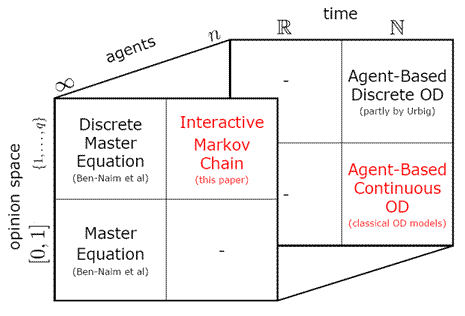Figure 1. Different approaches to model and analyse continuous opinion dynamics.

###The agent-based bounded confidence model of Hegselmann and Krause

2.1
We will start our analysis with a review about the agent-based bounded confidence model.

#### Definition of the HK process of opinion dynamics

2.2
Consider n agents and a discrete time step t. The opinion of agent i ∈ {1,...,n} at time t is the real number xi. We call the n-dimensional real column vector x(t) an opinion profile at time step t. The positive real number ε is called a bound of confidence.

2.3
For an opinion profile x and a bound of confidence ε we define the confidence matrix A as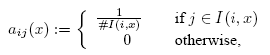with I(i,x) = {j with |xj - xi| ≤ ε}. (# is the number of elements of a set.)

Given an initial opinion profile x(0) we define the HK process of opinion dynamics as a sequence of opinion profiles (x(t))t recursively defined through x(t+1) = A(x(t)) x(t).

2.4
The heuristics of the HK process of opinion dynamics is that each agent moves to the arithmetic mean of all agents with opinion within his bound of confidence.

#### Dynamics in the HK model

2.5
It can be shown analytically that every HK process of opinion dynamics converges in finite time to a stabilised opinion profile of opinion clusters (Hegselmann and Krause (2002), and Lorenz (2005) for a bigger class of models). A cluster is a group of agents with the same opinion which are far enough from other agents to be uninfluenced of them. The most interesting parameter is, the number of clusters after stabilisation. For a given initial profile it obviously depends very much on the bound of confidence ε. But it is also very sensitive to the initial profile itself. There are some sufficient conditions under which consensus will be reached, but there is no direct argument what determines the number of clusters from the initial profile.

2.6
Thus, simulation has to play its role (Hegselmann and Krause (2002) gives an overview). The most simulated initial profiles contain only opinions between 0 and 1. On the one hand one studies the equidistant profile with opinions xi = (i-1)/(n-1) and on the other hand profiles with random and equally distributed opinions within [0,1]. Roughly, we can summarise that there are ε-phases where a certain number of clusters is most likely to emerge. The general trend is of course: The lower epsilon the higher the number of emerging clusters. But nevertheless very often a certain random initial profile leads to less clusters for a slightly lower epsilon.

2.7
It is easy to see: If we scale the initial profile by the real factor λ and the bound of confidence by |λ|, the emerging stabilised profile is the stabilised profile of the unscaled initial profile scaled by λ. Further on, if we translated all initial opinions by the same real value a, the emerging stabilised opinions are the stabilised opinions of the untranslated initial opinions translated by a. Thus, in simulations for equidistant or random initial profiles the relevant parameter that determines roughly the number of emerging clusters is the proportion of the bound of confidence at the range of initial opinions ε/(max xi - min xi), which we call ε/range. It is important to notice that because we will not use the same range of opinions in the following. To compare the results with former results of Hegselmann and Krause and the model of Weisbuch et al we have to compare ε/range.

#### The role of the number of agents

2.8
If we assume either equidistant or random distributions of opinions in the initial profile the number of agents n determines a whole opinion profile. Its influence is not clear. In a footnote Hegselmann (2004) conjectured that for every bound of confidence there might be a number of agents n for which an equidistant opinion profile xi = (i-1)/(n-1) leads to consensus. This conjecture may be inspired by a phenomena like in figure 2. Where raising the number of agents leads under constant ε/range to consensus.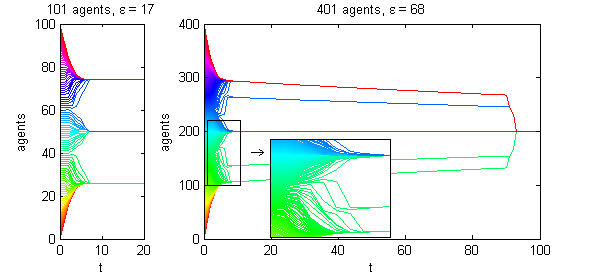Figure 2. Two processes with equidistant initial profiles and both with ε/range = 0.17. (The matlab m-file that generates the figure is fig2.m.)

2.9
Figure 2 suggests that the convergence to consensus is produced by something going on in the center. During the building of three big blocks of agents at time step eight, two small groups of agents survive. Each between one extreme and the central block. This constellation is a metastable state because it looks stable, but leads to very slow convergence to consensus.

2.10
The dynamics in an HK process of opinion dynamics starts at both extremes, while in the center nothing happens due to the uniform distribution of opinions. To get clear about the basic dynamics it is useful to study the dynamics onesided (figure 3). Thus, we regard an infinitely number of agents with equidistant opinions: the natural numbers including zero. In simulation we observe the formation of a cluster up to ε = 500. If Hegselmann's conjecture is right there must be an ε for which we observe no emergence of a cluster.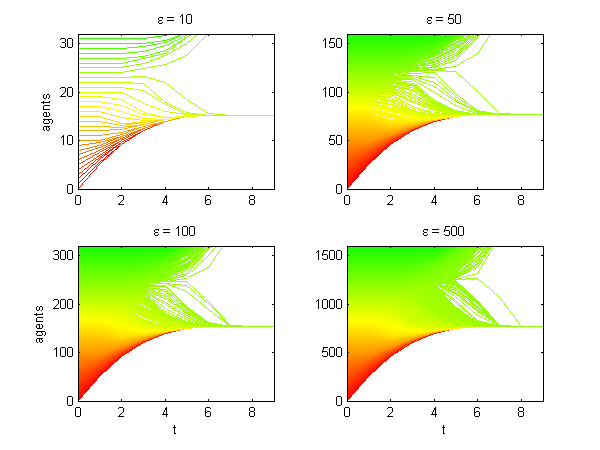Figure 3. Onesided dynamics in a HK process of opinion dynamics with the natural numbers including zero as initial profile. We observe the emergence of a cluster for different values of ε in a very (but not totally) similar manner after at least eight time steps. (matlab-code of the figure: fig3.m)

2.11
We study the onesided dynamics on a longer time scale (figure 4) and observe the formation of one cluster after another roughly in quite regular manner. But a detailed look shows that each emergence of a cluster differs slightly from the others and there are no regularities in the time steps when they emerge, the number of agents per cluster and the distances to the former cluster. This unregularities result not only from possible floating point errors but also from the nonlinear nature of the bounded confidence assumption.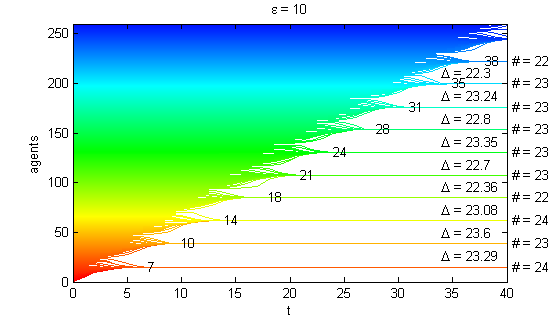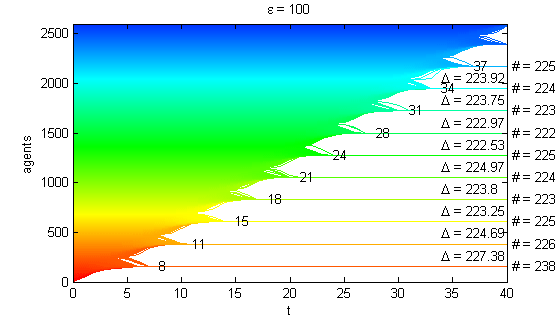Figure 4. Clustering in onesided dynamics in a HK process of opinion dynamics with the natural numbers including zero as initial profile for ε = 10 and 100. There is numerical information in the graphics about the time step when a cluster is complete, the number of agents in a cluster (#) and the distance to the former cluster (Δ). (matlab-code for the figure: fig4.m)

2.12
The simulation of onesided HK processes of opinion dynamics gives no hint on the truth of Hegselmann's conjecture, but it does not disprove it. In the next section we will analyse the HK model from the IMC perspective.

###Interactive Markov chain

#### Definition and interpretation of the HK interactive Markov chain

3.1
Assume a population of agents, each of which has one of the opinions {0, 1, ..., n}. The non-negative real row vector p(t) = [p0 p1 ... pn] is called opinion distribution at time step t. The value pi / ∑ pi represents the percentage of the population with opinion i. Thus, the population is represented by their distribution to opinion classes and not by individual agents anymore. That is why we can speak with some legitimation about an infinite population.

3.2
Let the natural number k be the discrete bound of confidence. Then we define the k-local mean of opinion class i with respect to p as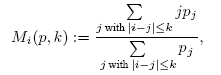for a positive denominator. If the denominator is zero we define Mi(p,k) = i. We should define pi = 0 for all i ∉ {0, 1, ..., n} for convenience.

With abbreviation Mi = Mi(p,k) we define the HK transition matrix B(p,k) as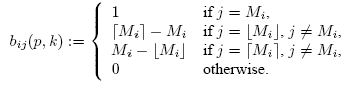3.3
The brackets ⌈ ⌉ define rounding to the upper integer and ⌊ ⌋ rounding to the lower integer. As always in a Markov chain the entry bij stands for the fraction of agents that moves from state i to state j. The above setup stands for the heuristics that all agents of opinion class i move to the opinion class of the k-local mean of i. If this is not an integer (as it happens nearly always), the population splits to the lower and the upper integer of the k-local mean. The closer the k-local mean is to one integer, the greater is the fraction that moves to that integer.

3.4
For an initial opinion distribution p(0) the interactive HK Markov chain is defined as a sequence of opinion distributions (p(t))t recursively defined through p(t+1) = p(t) B(p(t),k). The Markov chain is called interactive because the transition matrix depends on the actual distribution.

3.5
Notice that an opinion profile is a column vector, while an opinion distribution is a row vector. This is for matrix theoretical reasons: the confidence matrix and the transition matrix are both row-stochastic.

3.6
To compare the results of the IMC with the ABM, we want to point out the analogy ε/range ↔ k/n. Thus, we expect in the limit case of infinite numbers of agent and classes that dynamics are equal if ε/range = k/n.

3.7
On the way to the limit case we may see the number of classes as a measure for the accuracy how fine a continuous opinion can be expressed. This aspect has been studied by Urbig (2003) in the agent-based case.

#### Dynamics in the HK interactive Markov chain

3.8
The first thing to mention is that the HK interactive Markov Chain converges to a stabilised distribution in finite time within all our simulation setups. A formal proof of stabilisation is lacking. Further on in a stabilised distribution clusters appear as adjacent pairs of opinion classes, which lie at least k classes away from all other non-zero opinion classes. It is easy to see that such a pair which is not influenced by other classes with non-zero population will keep stable. Theoretically a cluster can consist of only one opinion class, but this happens for most initial distribution only in the center if we have an odd number of opinion classes.

3.9
We will focus on uniform initial distributions with pi = 1 for all i. To some extend this distribution is in analogy to the randomly distributed and the equidistant opinion profile with an infinite number of agents.

3.10
Figure 5 shows the dynamical behavior. We choose k/n = 0.16 ≈ 0.17 = ε/range in figure 2 and observe the same dynamics: We reach a meta-stable distribution with three big groups and two small groups of agents after eight time steps, which converges very slowly to consensus.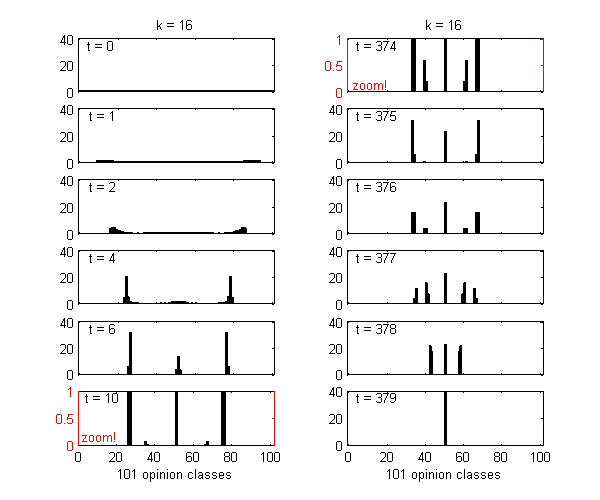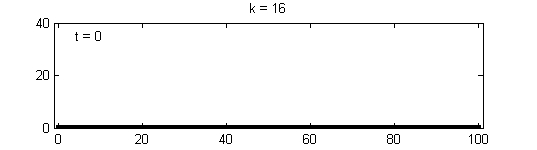Figure 5. HK interactive Markov chain with n = 100, k = 16, k/n = 0.16 and uniform initial distribution pi = 1. For interesting time steps and as complete animation. (matlab-code of the figure: fig5.m.)

3.11
Analogue to the ABM we study the onesided dynamics (like in figure 3) especially to check Hegselmann's conjecture from the IMC point of view. Figure 6 shows the first seven time steps for a onesided dynamic for k = 10 and k = 500. We observe the very similar formation of a cluster.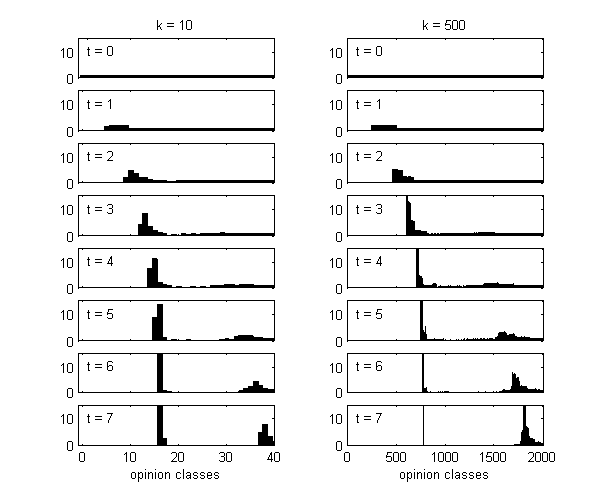Figure 6. Onesided HK interactive Markov chain for k = 10 and 500 with uniform initial distribution pi = 1 for all i = 0, 1, ... (matlab-code of the figure: fig6.m)

###Comparison between IMC and ABM

4.1
To compare the results about the formation of the first cluster in a onesided dynamic in the agent-based model and in the interactive Markov chain, we calculate the location of the first cluster for: ε = 10, 20, ..., 500 with initial profile the natural numbers including zero and k = 10, 20, ..., 500 with initial uniform distribution with the natural numbers including zero as opinion classes. (The location of a cluster in the agent-based model is the opinion of all agents in the cluster. The location of a cluster in the interactive Markov chain is the local mean of the two opinion classes it contains.) Figure 7 shows the normalised results (location of first cluster)/k respectively (location of first cluster)/ε.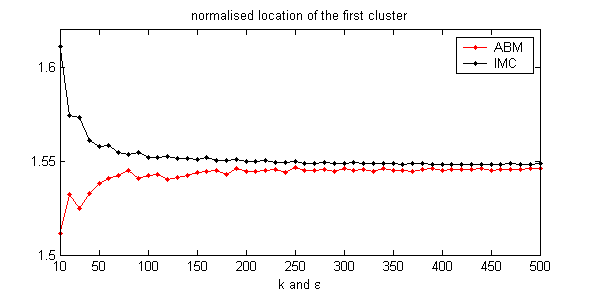Figure 7. Bound of confidence ε and discrete bound of confidence k vs. the normalised location of the first cluster (location of first cluster)/k and (location of first cluster)/ε.

4.2
The normalised locations of the first cluster seem to converge to the same fixed value for the ABM and the IMC, while the agent-based model is always lower than the interactive Markov chain. This leads to the hypothesis that the location of the first cluster in the interactive Markov chain is an upper bound for the location of the first cluster in the agent-based model. Further on the observed convergence-like behavior leads to the hypothesis, that Hegselmann's conjecture is wrong. But this is not a formal proof.

4.3
For investigation of the interactive Markov chain on a longer time scale analog to figure 4, we compute figure 8.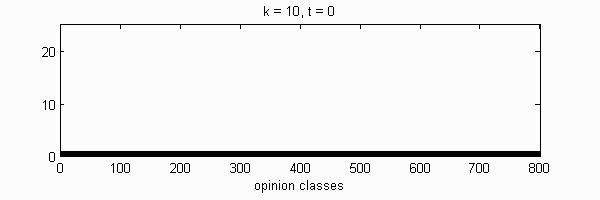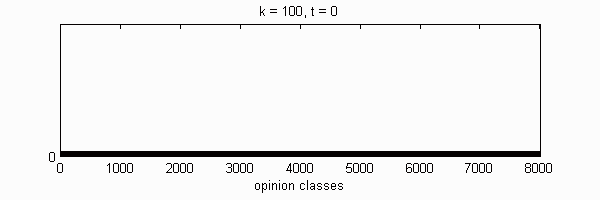Figure 8. Animation of onesided interactive Markov chains with uniform initial distribution (pi = 1 for all i = 0, 1, ...). Calculation for k = 10 and 100 until time step 100. (matlab-code of the figure similiar to fig6.m)

4.4
We observe very similar clustering dynamics for k = 10 and k = 100. To compare the results with the numerical data of figure 4 we give the same numerical data in table 1: time step when a cluster has formed, distance to the former cluster, number of agents in cluster (= sum of the population in the two opinion classes of the cluster).
Cluster 1 2 3 4 5 6 7 8 9 10
t k = 10 8 11 15 18 21 24 27 31 34 37
k = 100 8 12 15 18 21 25 28 31 34 37
Δ k = 10 - 24.41 23.74 23.45 23.38 23.29 23.21 23.16 23.16 23.08
k = 100 - 228.86 225.33 222.56 221.73 220.65 221.95 220.35 219.64 219.70
# k = 10 24.93 24.11 23.64 23.40 23.35 23.31 23.18 23.10 23.22 23.08
k = 100 238.84 227.48 224.91 222.57 220.90 220.50 223.13 220.75 216.69 220.87

Table 1. Numerical data for the first ten clusters of figure 8.

4.5
Further on figure 8 explains a reason why we observe in both models quite regular cluster formation from a global point of view, but also heavy and not regular variations in detail. This is due to the fact that indeed the major changes occur at the position where a cluster is forming, but every formation of a cluster causes some very small changes in the uniform distribution for the opinion classes with higher numbers. This small changes carry forward quicker than the major changes and get slowly bigger, as we can observe in figure 8. We have an evolving small dynamic running in front of the major dynamic. Thus each cluster formation occurs in a similar manner but with some influence from the small changes in the upper classes.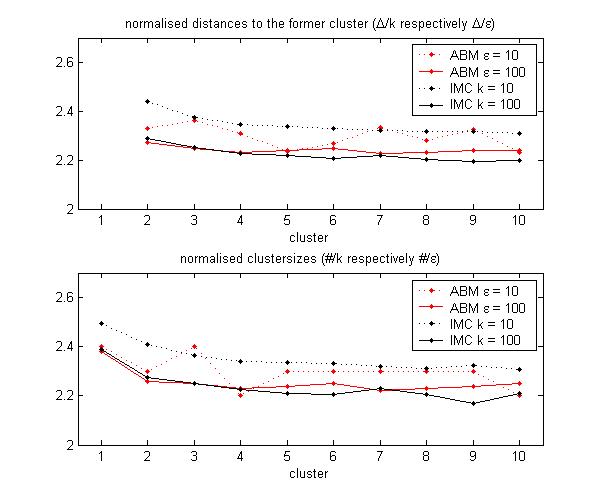Figure 9. Normalised data of figure 4 and table 1.

4.6
Figure 9 collects data about the first ten clusters in a onesided opinion dynamic from figure 4 and table 1. The data is normalised so that we can compare ABM and IMC and ε, k = 10 and 100. We observe that the IMC is not an upper bound for further clusters as it is in figure 7 for the location of the first cluster. We observe that higher ε, k leads to a little lower values and also to a lower distance between ABM and IMC.

4.7
In this section we saw that the interactive Markov chain captures the same dynamics as the agent-based model and may be regarded as a limit model for infinitely many agents. In the next section we will compute bifurcation diagrams to analyse the dynamics of the ε/range respectively k/n in a systematic way.

###Bifurcation Diagrams

5.1
The most studied parameter in continuous opinion dynamics under bounded confidence is the bound of confidence ε. While Krause and Hegselmann (2002, e.g. figure 3) study added stabilised profiles of a sample of several random initial profiles, Weisbuch et al (2002, figure 3) focussed on the number of evolving clusters also for a sample of random initial profiles. Both studies and visualisations have disadvantages. First, the stabilised profile is heavily dependent on the initial profile, thus both studies show the underlying dynamics only in a noisy way. Further on both visualisations capture only a part of the dynamical behavior. While the added stabilised profiles focus on the positions of the opinions after stabilisation it does not show the number of evolving clusters in a clear way. The other way round the Weisbuch et al graphic does not capture the locations and sizes of the clusters.

5.2
The interactive Markov chain gives the chance to study random (and equally distributed) initial profiles in total with one uniform opinion distribution. The results should hold also hold for the ABM in the limit case for a great number of agents. But they also show a kind of attractive behavior for lower numbers of agents.

We calculate the interactive Markov chains until stabilisation for the uniform initial distribution and all interesting discrete bounds of confidence. Figure 10 shows the results for n = 100 and k = 1, ..., 30 in an animation stabilising in a kind of bifurcation diagram.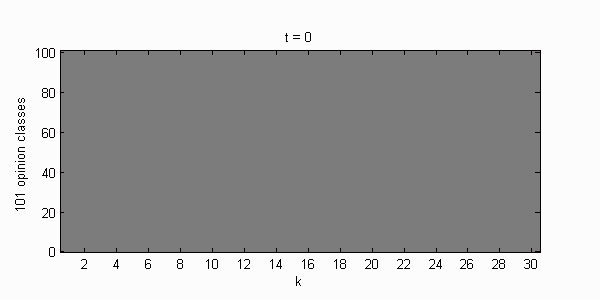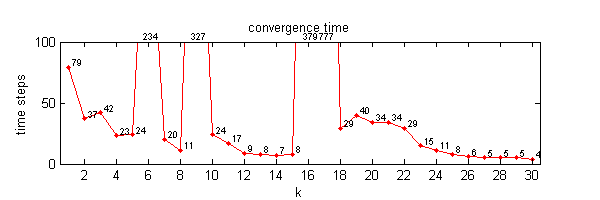Figure 10. Animation of the interactive Markov chains with uniform initial distribution for n = 100 and k = 1, ..., 30 and convergence time for stabilisation. The gray scale symbolises the magnitude of population in the opinion class: white is zero, black is great magnitude. (matlab-code of the figure: fig10.m)

5.3
While figure 10 is discrete by view, we will give in figure 11 the same bifurcation diagram for n = 1000 and k = 1,...,300 to get closer to the continuous opinion and continuous ε in the ABM.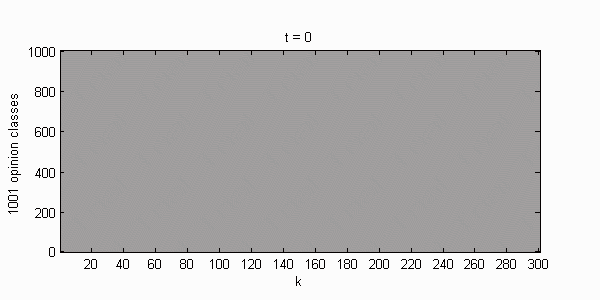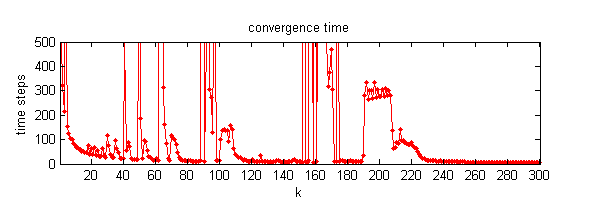Figure 11. Animation of the interactive Markov chains with uniform initial distribution for n = 1000 and k = 1, ..., 300 and convergence time for stabilisation. The gray scale symbolises the magnitude of population in the opinion class: white is zero, black is great magnitude. (matlab-code similar to fig10.m)

5.4
We call the stabilised figures 10 and 11 bifurcation diagrams according to the theory of dynamical systems. Where a bifurcation is a change in the structure of the attractor of a system regarding a parameter. In our setting we define a bifurcation as a change of the number of opinions of the stabilised distribution regarding k.

5.5
If we see a bifurcation as an increase of opinions (as the period-doubling phenomena in chaos theory) we have to read our bifurcation diagrams from right to left. We will describe the bifurcations of the system in figure 11 in this way in the following. To compare the results of the two diagrams and the results about the agent-based models we will refer the normalised values ε = k/n.

5.6
For ε = 0.3 we have on central cluster. Going down with ε we observe the bifurcation into three clusters (one small central and two big off-central) at ε = 0.19. The small central cluster grows slowly, but at 0.174 consensus strikes back. In an ε-phase from 0.174 to 0.152 we have an unordered switching between consensus and three clusters (with growing central cluster). Consensus in this phase is only reached due to very slow convergence like in figure 2 and 5. The consensus strikes back phase ends at ε = 0.152 with a stabilisation to three clusters again, while the central cluster is growing. At ε = 0.1 the central cluster bifurcates again in the same way but on a shorter scale from ε = 0.1 to 0.069. We can assume repetitions of the bifurcation of the central cluster on every time shorter scales in the same way, but the accuracy of the data does not allow us to observe further bifurcations in a clear way.

5.7
For a brief comparison with the agent-based model we give the same diagrams for the equidistant initial profiles of 101 agents (figure 12) and 1001 agents (figure 13). While 101 agents do not show a consensus strikes back phase, 1001 agents show it. But notice that the diagram for 1001 agents shows some asymmetries, which are not possible due to theoretical reasons, this is caused by numerical vulnerability of the process.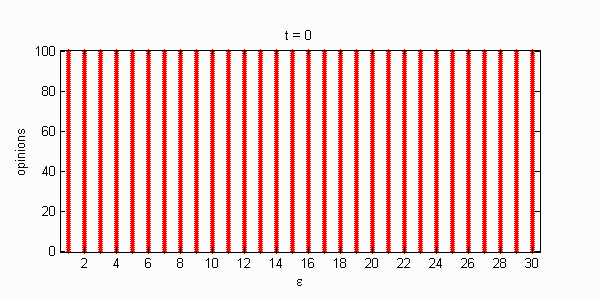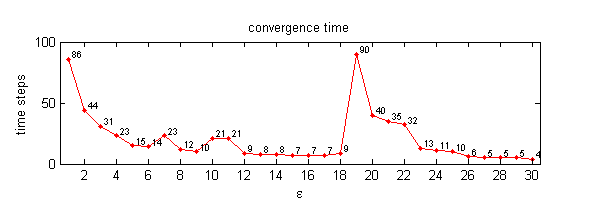Figure 12. Animation of the agent-based model with equidistant initial distribution of 101 opinions for bound of confidence ε = 1, ..., 30 and convergence time for stabilisation. (matlab-code of the figure: fig12.m)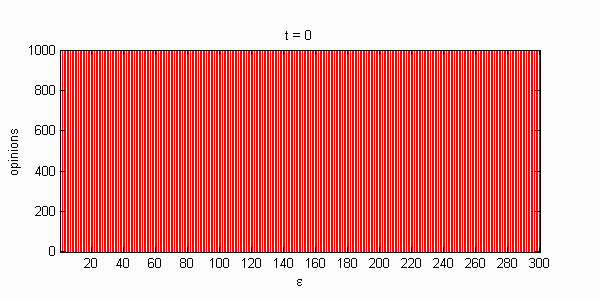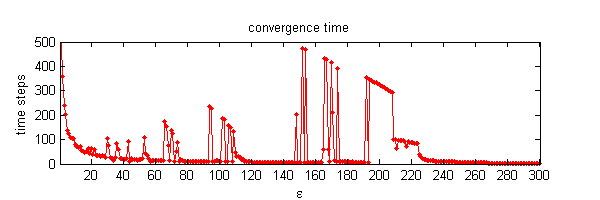Figure 13. Animation of the agent-based model with equidistant initial distribution of 1001 opinions for bound of confidence ε = 1, ..., 300 and convergence time for stabilisation. (matlab-code similar to fig12.m)

5.8
The bifurcation diagrams of the interactive Markov chain 10 and 11 should be regarded more general than figure 12 and 13, because they capture all agent-based processes with equally distributed and sufficiently enough agents.

###Conclusion

6.1
We have seen that the IMC is in pretty good analogy with the ABM not only by its heuristics but also in simulation. Due to the globalisation to the population level with the IMC some further insights were possible.

6.2
Random equally distributed initial profiles lead for great numbers of agents systematically to parameter regions of metastable states where convergence to consensus lasts very long. There seems to be no upper bound for the convergence time with rising number of agents. The problem of metastable states is also getting worse in the IMC for a rising number of opinion classes.

6.3
We should expect a weaker trend to metastable states with long convergence times for not uniform distributions. But starting with a random sample must by far not capture the full dynamical possibilities of a system. A systematic characterisation of other initial distributions and an analysis about the effects is a challenge for future research.

6.4
The metastable states lead to the surprising phenomenon that consensus strikes back after polarisation for lower bounds of confidence. It can be explained by a small hill that we can observe in figure 6 for k = 500 and time step t = 4. The hill is in front of the forming cluster and vanishes in the following time steps. But if two clusters arrive from both sides they get in contact with the central cluster and thus create the metastable state. It would be very interesting to analyse why this small hill forms.

6.5
The Hegselmann conjecture that enough agents force consensus seems to be wrong. It might be true that the first bifurcation at ε = 0.19 might happen for successively lower values such that it may disappear and further on it might be possible that the consensus strikes back phase gets stable (without the switching to three clusters) but at least the bifurcation into the three stable clusters will probably appear because we saw cluster formation in the onesided analysis. Nevertheless the Hegselmann conjecture is not disproved by this paper.

6.6
The clustering in the HK model have shown to be relatively systematic by global view but chaotic in the details of the dynamics. This is especially interesting because the chaotic-like behavior is not caused by random sampling. None of our graphics depends on random samples, the chaotic fluctuations evolve from regular initial conditions due to the dynamic itself .

6.7
Further on there is no formal proof of stabilisation for the IMC which would be very interesting to understand the driving forces of stabilisation and clustering on a more general level.

6.8
We have not studied the effects of the accuracy in the IMC which would be another point of interest in future research.

6.9
It is also possible to formulate an IMC for the model of Weisbuch et al, there is a first comparison of the IMC for the two models in Lorenz (2005).

6.10
Overall we have seen that the Hegselmann-Krause model has some interesting features even in the basic model without extensions. Metastable opinion configurations and small opinion groups that convince others to consensus are features that can be linked directly to compromising under bounded confidence. Further on we have seen that we can not argue, that higher confidence leads automatically to less opinion clusters.

###References

AMBLARD, F. and DEFFUANT, G. (2004), The role of network topology on extremism propagation with the relative agreement opinion dynamics, Physica A, vol. 343, 725-738

BEN-NAIM, E., KRAPIVSKY, P.L. and REDNER S. (2003), Bifurcation and Patterns in Compromise Processes, Physica D, vol. 183, 190-204

CONLISK, J. (1976), Interactive Markov Chains, Journal of Mathematical Sociology, vol. 4, 157-185

DEFFUANT, G., NEAU, D., AMBLARD, F. and WEISBUCH, G. (2002), How can extremism prevail? A study based on the relative agreement interaction model, Journal of Artificial Societies and Social Simulation, vol. 5, no. 4, https://www.jasss.org/5/4/1.html

DITTMER, Jan Christian (2001), Consensus Formation under bounded Confidence, Nonlinear Analysis, vol. 47, 4615-4621

HEGSELMANN, R. (2004). Opinion Dynamics ñ Insights by Radically Simplifying Models,.In: Gillies D. (Ed), Laws and Models in Science, London, 1-29.

HEGSELMANN, R. and KRAUSE, U. (2002) Opinion Dynamics and Bounded Condence Models, Analysis and Simulation, Journal of Artificial Societies and Social Simulations, vol. 5, no. 3, https://www.jasss.org/JASSS/5/3/2.html

HERMANNS, H. (2002), Interactive Markov chains: and the quest for quantified quality, Springer

HORST, U. (2001), Convergence of locally and globally interacting Markov chains, Stochastic Processes and their Application, vol. 96, 99-121

JAGER, W. and AMBLARD, F. (2005), Uniformity, bipolarisation and pluriformity captured as generic stylized behaviour with an agent-based simulation model of attitude change, Computational and Mathematical Organization Theory, vol. 10, 295-303

LORENZ, J. (2005), A Stabilization Theorem for Dynamics of Continuous Opinions, Physica A, Vol. 355 (1), 217-223

LORENZ, J. (2005), Continuous Opinion Dynamics: Insights through Interactive Markov Chains; In Proceedings of IASTED Conference "Modelling, Simulation and Optimization ~MSO 2005~", August 29-31, 2005, Oranjestad, Aruba available at http://www.janlo.de

NEAU (2000), D. Révisions des croyances dans un systéme díagents en interaction, rapport díoption de líécole polytechnique, available at http://www.lps.ens.fr/~weisbuch/rapneau.ps

POLHILL, G. and IZQUIERDO, L. (2005), three papers in JASSS

URBIG, D. (2003), Attitude Dynamics with Limited Verbalisation Capabilities, Journal of Artificial Societies and Social Simulation, vol. 6, no. 1, https://www.jasss.org/6/1/2.html

URBIG, D. and LORENZ, J. (2004), Communication regimes in opinion dynamics: Changing the number of communicating agents; Proceedings of the Second Conference of the European Social Simulation Association (ESSA), Valladolid, Spain, September 16-19, 2004, available at http://www.janlo.de

WEISBUCH, G., DEFFUANT, G., AMBLARD, F. and NADAL, J. (2002), Meet, Discuss and Segregate!, Complexity, vol. 7 no. 3, 55-63